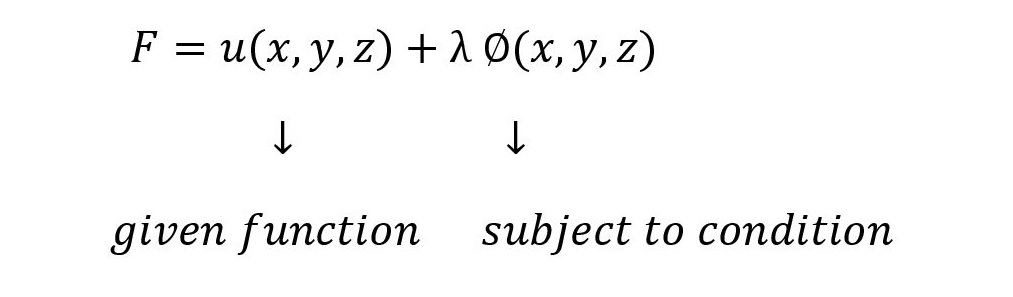## LaGrange’s method of multipliers with one subsidiary condition

### Step 1:

Auxilliary function is formed by### Step 2:

Form equations
Fx=0 ,Fy=0 and Fz=0 where
Fx is the partial derivative of ‘F’ with respect to ‘x’
Fy is the partial derivative of ‘F’ with respect to ‘y’ and
Fz is the partial derivative of ‘F’ with respect to ‘z’.

### Step 3:

Solve for (x,y,z) and ‘λ’,the values of u(x,y,z)are the Stationary values.